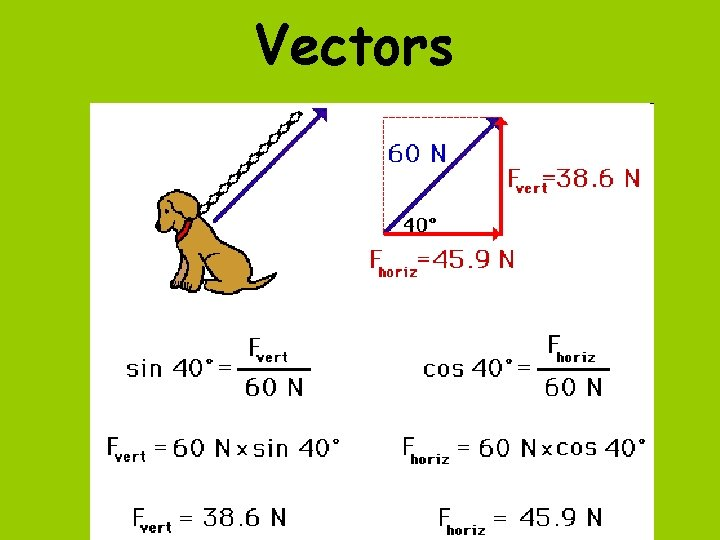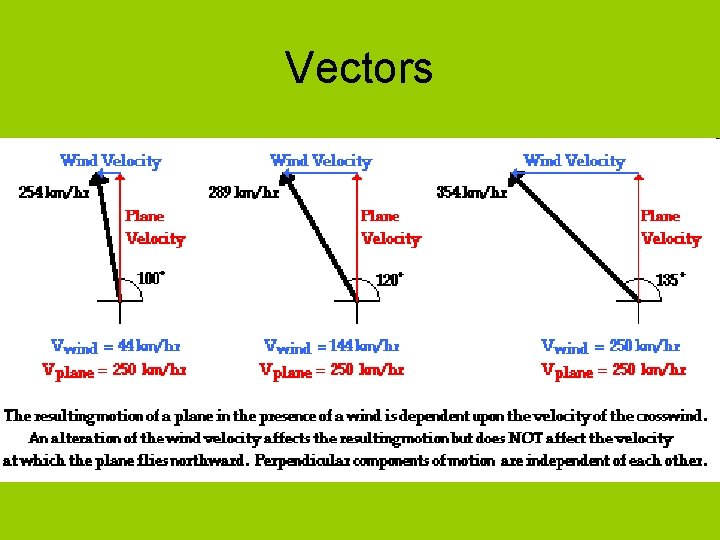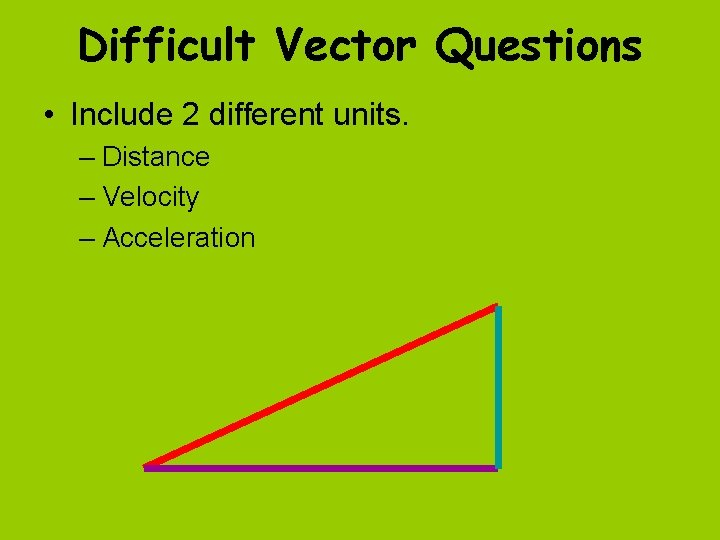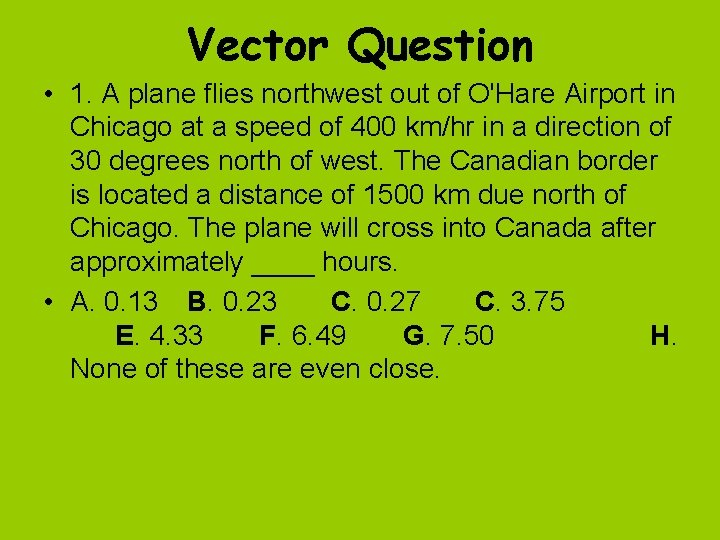# Vectors Vectors Vectors Vectors Distance Velocity Acceleration X

• Slides: 8VectorsVectorsVectorsVectors • Distance • Velocity • Acceleration X and Y Vx Vy Ax AyDifficult Vector Questions • Include 2 different units. – Distance – Velocity – AccelerationVector Question • 1. A plane flies northwest out of O'Hare Airport in Chicago at a speed of 400 km/hr in a direction of 30 degrees north of west. The Canadian border is located a distance of 1500 km due north of Chicago. The plane will cross into Canada after approximately ____ hours. • A. 0. 13 B. 0. 23 C. 0. 27 C. 3. 75 E. 4. 33 F. 6. 49 G. 7. 50 H. None of these are even close.Answer • The plane has both a northward and a westward motion. • The northward motion towards the Canadian border is dependent upon the component of velocity in the northern direction. • To solve this problem, the northern component of the plane's velocity must first be determined.Solution • VNorth = 400 • sin (150 degrees) = 200 km/hr • Once determined, the d = v • t equation can be used to determine the time that it takes the plane to reach the Canadian border. • DNorth = VNorth • t t = DNorth / VNorth = (1500 km) / (200 km/hr) = 7. 5 hr • Answer: G